Anzeige

# Semiconductors (rawat d agreatt)

12. Oct 2014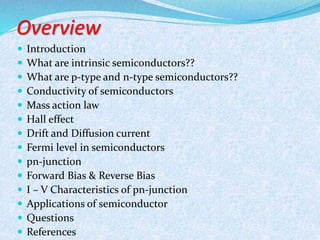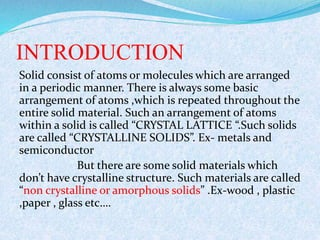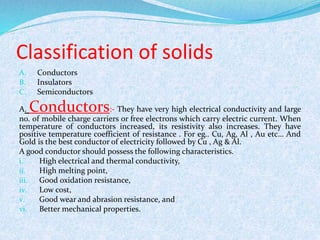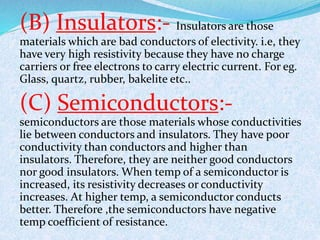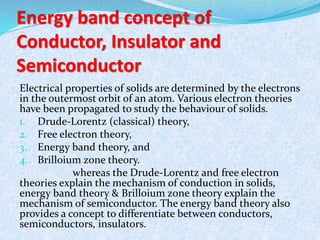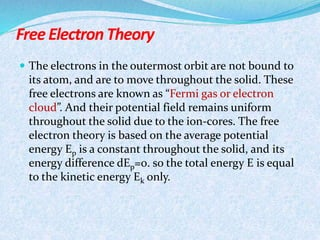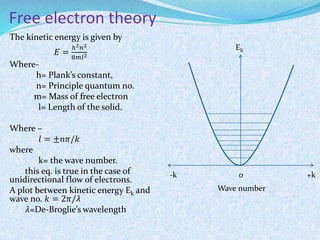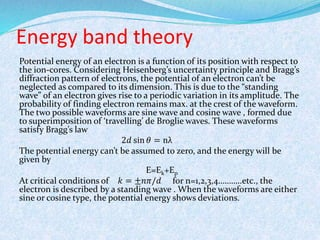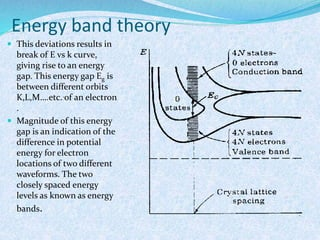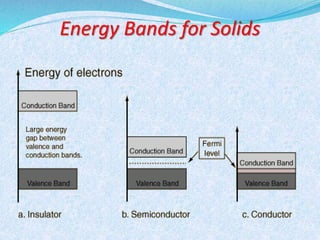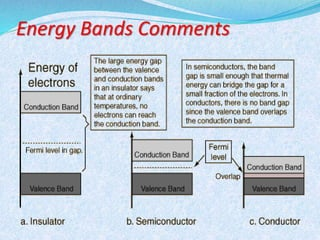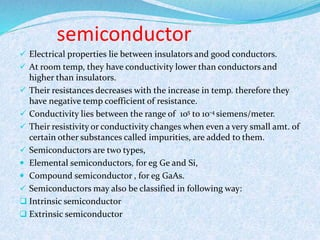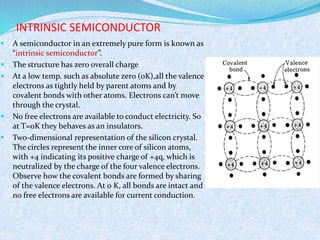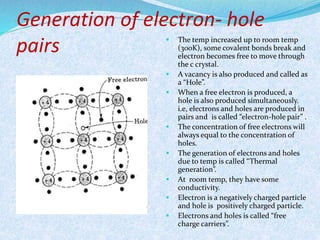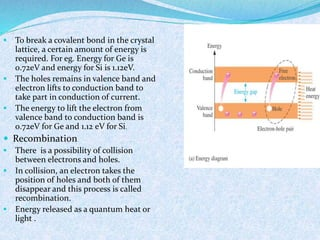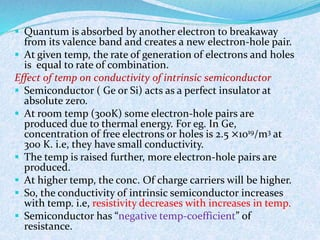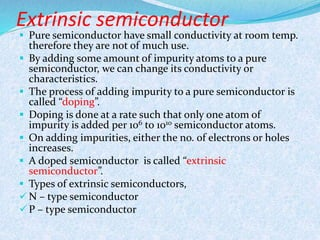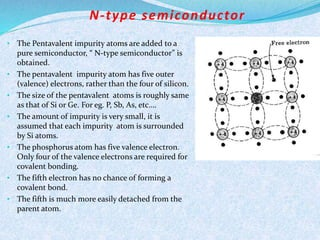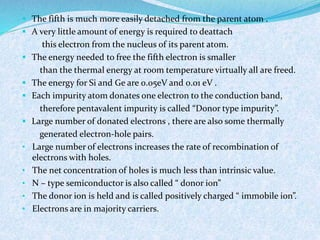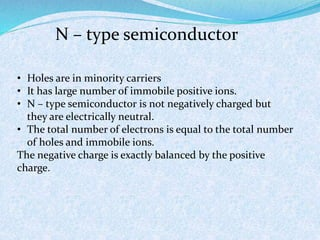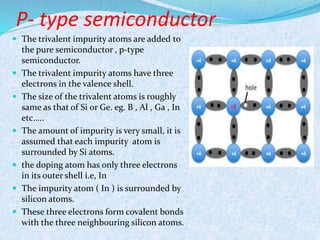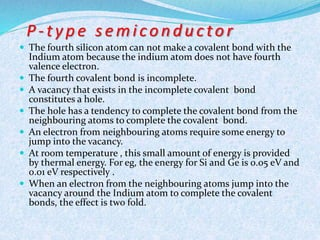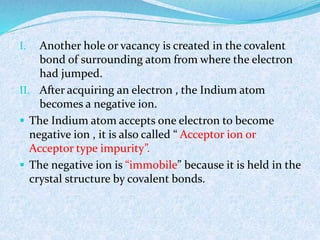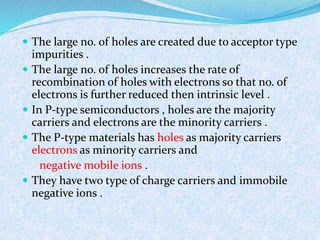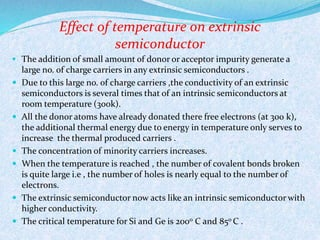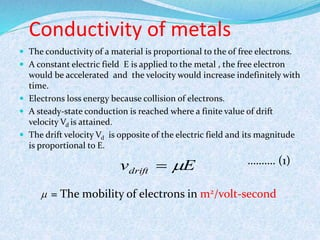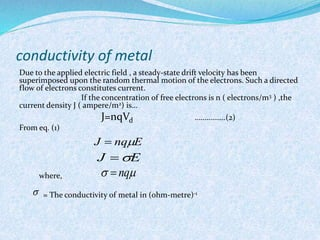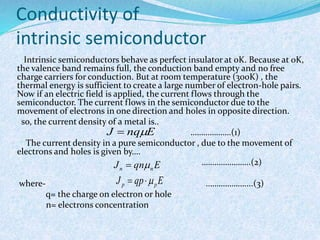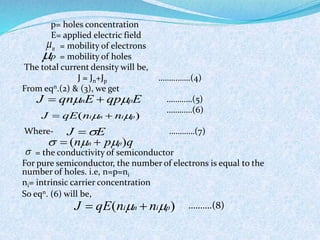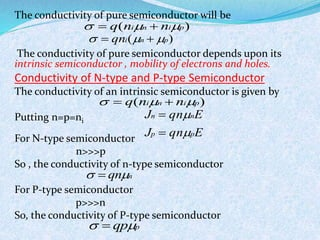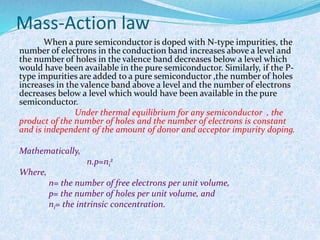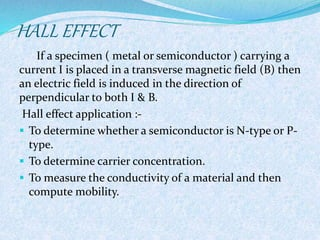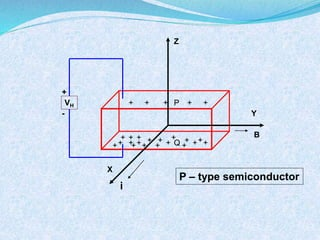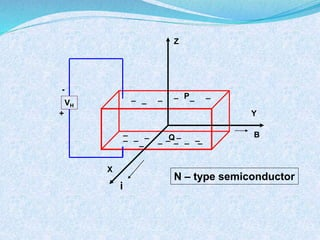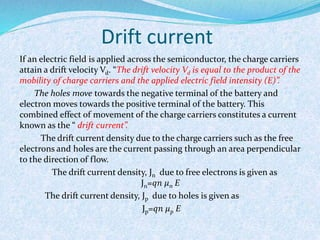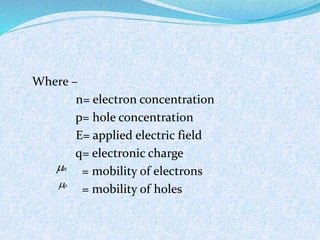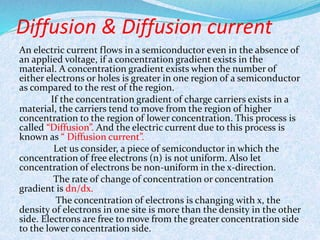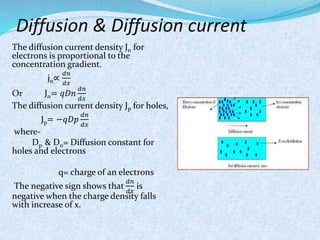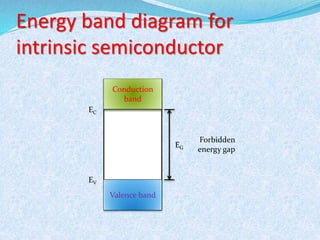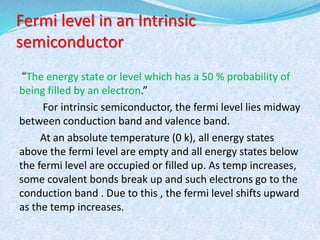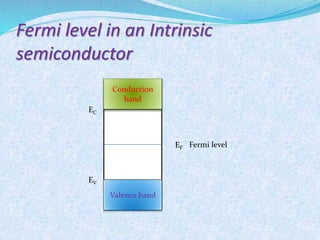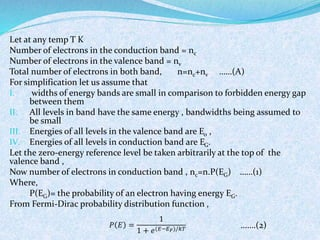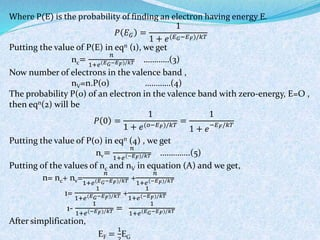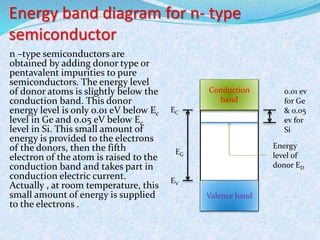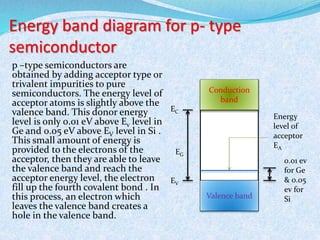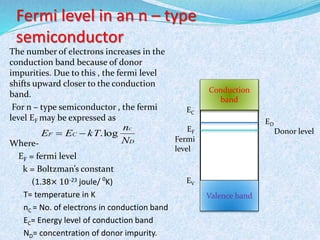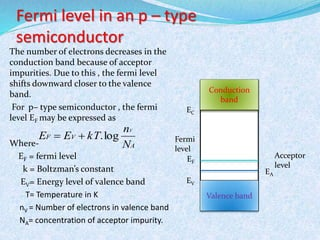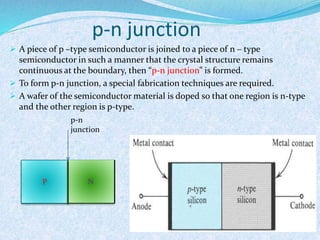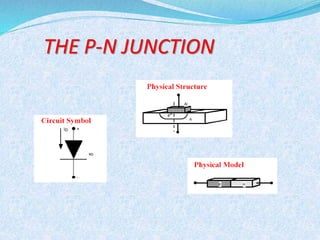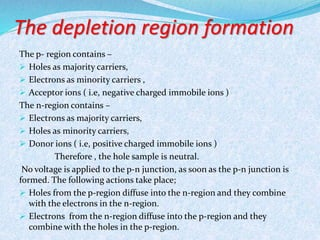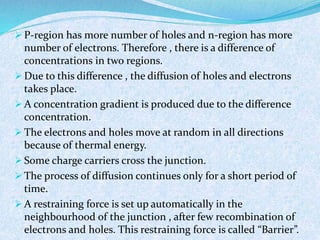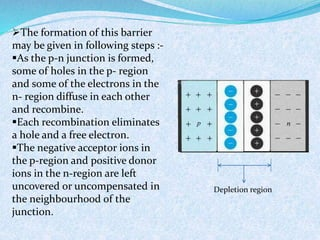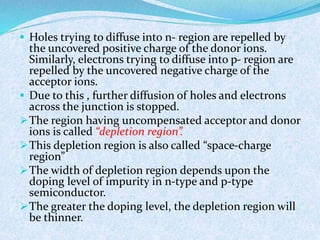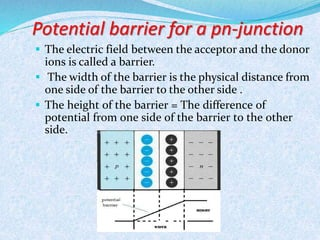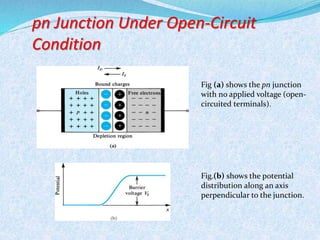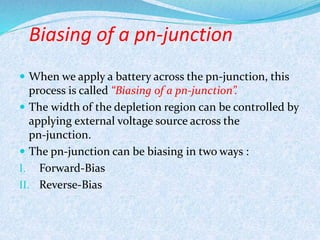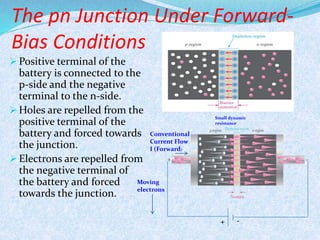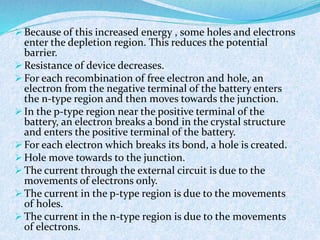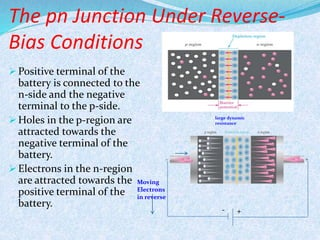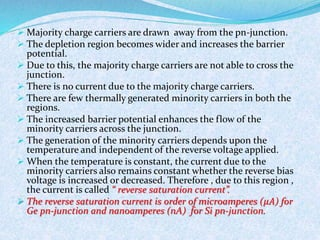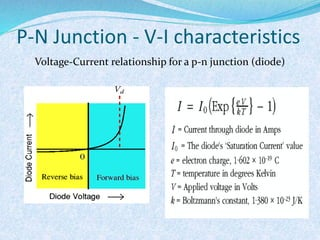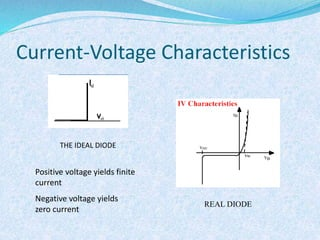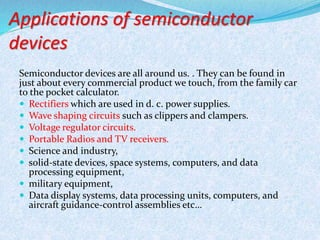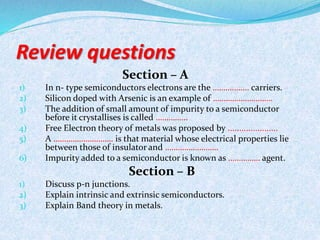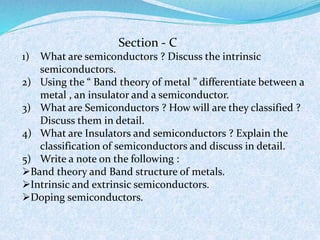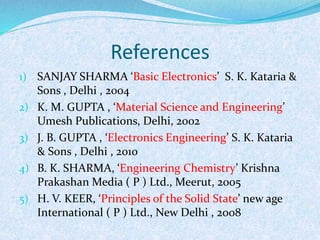1 von 68
Anzeige

### Semiconductors (rawat d agreatt)

1. Presentation by- Saurav k. Rawat (Rawat DA Greatt)
2. Overview  Introduction  What are intrinsic semiconductors??  What are p-type and n-type semiconductors??  Conductivity of semiconductors  Mass action law  Hall effect  Drift and Diffusion current  Fermi level in semiconductors  pn-junction  Forward Bias & Reverse Bias  I – V Characteristics of pn-junction  Applications of semiconductor  Questions  References
3. INTRODUCTION Solid consist of atoms or molecules which are arranged in a periodic manner. There is always some basic arrangement of atoms ,which is repeated throughout the entire solid material. Such an arrangement of atoms within a solid is called “CRYSTAL LATTICE “.Such solids are called “CRYSTALLINE SOLIDS”. Ex- metals and semiconductor But there are some solid materials which don’t have crystalline structure. Such materials are called “non crystalline or amorphous solids” .Ex-wood , plastic ,paper , glass etc….
4. Classification of solids A. Conductors B. Insulators C. Semiconductors A.Conductors:- They have very high electrical conductivity and large no. of mobile charge carriers or free electrons which carry electric current. When temperature of conductors increased, its resistivity also increases. They have positive temperature coefficient of resistance . For eg.. Cu, Ag, Al , Au etc… And Gold is the best conductor of electricity followed by Cu , Ag & Al. A good conductor should possess the following characteristics. i. High electrical and thermal conductivity, ii. High melting point, iii. Good oxidation resistance, iv. Low cost, v. Good wear and abrasion resistance, and vi. Better mechanical properties.
5. (B) Insulators:- Insulators are those materials which are bad conductors of electivity. i.e, they have very high resistivity because they have no charge carriers or free electrons to carry electric current. For eg. Glass, quartz, rubber, bakelite etc.. (C) Semiconductors:- semiconductors are those materials whose conductivities lie between conductors and insulators. They have poor conductivity than conductors and higher than insulators. Therefore, they are neither good conductors nor good insulators. When temp of a semiconductor is increased, its resistivity decreases or conductivity increases. At higher temp, a semiconductor conducts better. Therefore ,the semiconductors have negative temp coefficient of resistance.
6. Energy band concept of Conductor, Insulator and Semiconductor Electrical properties of solids are determined by the electrons in the outermost orbit of an atom. Various electron theories have been propagated to study the behaviour of solids. 1. Drude-Lorentz (classical) theory, 2. Free electron theory, 3. Energy band theory, and 4. Brilloium zone theory. whereas the Drude-Lorentz and free electron theories explain the mechanism of conduction in solids, energy band theory & Brilloium zone theory explain the mechanism of semiconductor. The energy band theory also provides a concept to differentiate between conductors, semiconductors, insulators.
7. Free Electron Theory  The electrons in the outermost orbit are not bound to its atom, and are to move throughout the solid. These free electrons are known as “Fermi gas or electron cloud”. And their potential field remains uniform throughout the solid due to the ion-cores. The free electron theory is based on the average potential energy Ep is a constant throughout the solid, and its energy difference dEp=0. so the total energy E is equal to the kinetic energy Ek only.
8. Free electron theory The kinetic energy is given by 퐸 = ℎ2푛2 8푚푙2 Where-h= Plank’s constant, n= Principle quantum no. m= Mass of free electron l= Length of the solid. Where – 푙 = ±푛휋/푘 where k= the wave number. this eq. is true in the case of unidirectional flow of electrons. A plot between kinetic energy Ek and wave no. 푘 = 2휋/휆 휆=De-Broglie’s wavelength Ek -k 0 +k Wave number
9. Energy band theory Potential energy of an electron is a function of its position with respect to the ion-cores. Considering Heisenberg’s uncertainty principle and Bragg’s diffraction pattern of electrons, the potential of an electron can’t be neglected as compared to its dimension. This is due to the “standing wave” of an electron gives rise to a periodic variation in its amplitude. The probability of finding electron remains max. at the crest of the waveform. The two possible waveforms are sine wave and cosine wave , formed due to superimposition of ‘travelling’ de Broglie waves. These waveforms satisfy Bragg’s law 2푑 sin 휃 = nλ The potential energy can’t be assumed to zero, and the energy will be given by E=Ek+Ep At critical conditions of 푘 = ±푛휋/푑 for n=1,2,3,4………..etc., the electron is described by a standing wave . When the waveforms are either sine or cosine type, the potential energy shows deviations.
10. Energy band theory  This deviations results in break of E vs k curve, giving rise to an energy gap. This energy gap Eg is between different orbits K,L,M….etc. . of an electron .  Magnitude of this energy gap is an indication of the difference in potential energy for electron locations of two different waveforms. The two closely spaced energy levels as known as energy bands.
11. Energy Bands for Solids
13. semiconductor  Electrical properties lie between insulators and good conductors.  At room temp, they have conductivity lower than conductors and higher than insulators.  Their resistances decreases with the increase in temp. therefore they have negative temp coefficient of resistance.  Conductivity lies between the range of 105 to 10-4 siemens/meter.  Their resistivity or conductivity changes when even a very small amt. of certain other substances called impurities, are added to them.  Semiconductors are two types,  Elemental semiconductors, for eg Ge and Si,  Compound semiconductor , for eg GaAs.  Semiconductors may also be classified in following way:  Intrinsic semiconductor  Extrinsic semiconductor
14. INTRINSIC SEMICONDUCTOR  A semiconductor in an extremely pure form is known as “intrinsic semiconductor”.  The structure has zero overall charge  At a low temp. such as absolute zero (0K),all the valence electrons as tightly held by parent atoms and by covalent bonds with other atoms. Electrons can’t move through the crystal.  No free electrons are available to conduct electricity. So at T=0K they behaves as an insulators.  Two-dimensional representation of the silicon crystal. The circles represent the inner core of silicon atoms, with +4 indicating its positive charge of +4q, which is neutralized by the charge of the four valence electrons. Observe how the covalent bonds are formed by sharing of the valence electrons. At 0 K, all bonds are intact and no free electrons are available for current conduction.
15. Generation of electron- hole pairs  The temp increased up to room temp (300K), some covalent bonds break and electron becomes free to move through the c crystal.  A vacancy is also produced and called as a “Hole”.  When a free electron is produced, a hole is also produced simultaneously. i.e, electrons and holes are produced in pairs and is called “electron-hole pair” .  The concentration of free electrons will always equal to the concentration of holes.  The generation of electrons and holes due to temp is called “Thermal generation”.  At room temp, they have some conductivity.  Electron is a negatively charged particle and hole is positively charged particle.  Electrons and holes is called “free charge carriers”.
16.  To break a covalent bond in the crystal lattice, a certain amount of energy is required. For eg. Energy for Ge is 0.72eV and energy for Si is 1.12eV.  The holes remains in valence band and electron lifts to conduction band to take part in conduction of current.  The energy to lift the electron from valence band to conduction band is 0.72eV for Ge and 1.12 eV for Si.  Recombination  There is a possibility of collision between electrons and holes.  In collision, an electron takes the position of holes and both of them disappear and this process is called recombination.  Energy released as a quantum heat or light .
17.  Quantum is absorbed by another electron to breakaway from its valence band and creates a new electron-hole pair.  At given temp, the rate of generation of electrons and holes is equal to rate of combination. Effect of temp on conductivity of intrinsic semiconductor  Semiconductor ( Ge or Si) acts as a perfect insulator at absolute zero.  At room temp (300K) some electron-hole pairs are produced due to thermal energy. For eg. In Ge, concentration of free electrons or holes is 2.5 ×1019/m3 at 300 K. i.e, they have small conductivity.  The temp is raised further, more electron-hole pairs are produced.  At higher temp, the conc. Of charge carriers will be higher.  So, the conductivity of intrinsic semiconductor increases with temp. i.e, resistivity decreases with increases in temp.  Semiconductor has “negative temp-coefficient” of resistance.
18. Extrinsic semiconductor  Pure semiconductor have small conductivity at room temp. therefore they are not of much use.  By adding some amount of impurity atoms to a pure semiconductor, we can change its conductivity or characteristics.  The process of adding impurity to a pure semiconductor is called “doping”.  Doping is done at a rate such that only one atom of impurity is added per 106 to 1010 semiconductor atoms.  On adding impurities, either the no. of electrons or holes increases.  A doped semiconductor is called “extrinsic semiconductor”.  Types of extrinsic semiconductors,  N – type semiconductor  P – type semiconductor
19. N- type semiconductor • The Pentavalent impurity atoms are added to a pure semiconductor, “ N-type semiconductor” is obtained. • The pentavalent impurity atom has five outer (valence) electrons, rather than the four of silicon. • The size of the pentavalent atoms is roughly same as that of Si or Ge. For eg. P, Sb, As, etc…. • The amount of impurity is very small, it is assumed that each impurity atom is surrounded by Si atoms. • The phosphorus atom has five valence electron. Only four of the valence electrons are required for covalent bonding. • The fifth electron has no chance of forming a covalent bond. • The fifth is much more easily detached from the parent atom.
20.  The fifth is much more easily detached from the parent atom .  A very little amount of energy is required to deattach this electron from the nucleus of its parent atom.  The energy needed to free the fifth electron is smaller than the thermal energy at room temperature virtually all are freed.  The energy for Si and Ge are 0.05eV and 0.01 eV .  Each impurity atom donates one electron to the conduction band, therefore pentavalent impurity is called “Donor type impurity”.  Large number of donated electrons , there are also some thermally generated electron-hole pairs. • Large number of electrons increases the rate of recombination of electrons with holes. • The net concentration of holes is much less than intrinsic value. • N – type semiconductor is also called “ donor ion” • The donor ion is held and is called positively charged “ immobile ion”. • Electrons are in majority carriers.
21. N – type semiconductor • Holes are in minority carriers • It has large number of immobile positive ions. • N – type semiconductor is not negatively charged but they are electrically neutral. • The total number of electrons is equal to the total number of holes and immobile ions. The negative charge is exactly balanced by the positive charge.
22. P- type semiconductor  The trivalent impurity atoms are added to the pure semiconductor , p-type semiconductor.  The trivalent impurity atoms have three electrons in the valence shell.  The size of the trivalent atoms is roughly same as that of Si or Ge. eg. B , Al , Ga , In etc…..  The amount of impurity is very small, it is assumed that each impurity atom is surrounded by Si atoms.  the doping atom has only three electrons in its outer shell i.e, In  The impurity atom ( In ) is surrounded by silicon atoms.  These three electrons form covalent bonds with the three neighbouring silicon atoms.
23. P - t y p e s emi c o n d u c t o r  The fourth silicon atom can not make a covalent bond with the Indium atom because the indium atom does not have fourth valence electron.  The fourth covalent bond is incomplete.  A vacancy that exists in the incomplete covalent bond constitutes a hole.  The hole has a tendency to complete the covalent bond from the neighbouring atoms to complete the covalent bond.  An electron from neighbouring atoms require some energy to jump into the vacancy.  At room temperature , this small amount of energy is provided by thermal energy. For eg, the energy for Si and Ge is 0.05 eV and 0.01 eV respectively .  When an electron from the neighbouring atoms jump into the vacancy around the Indium atom to complete the covalent bonds, the effect is two fold.
24. I. Another hole or vacancy is created in the covalent bond of surrounding atom from where the electron had jumped. II. After acquiring an electron , the Indium atom becomes a negative ion.  The Indium atom accepts one electron to become negative ion , it is also called “ Acceptor ion or Acceptor type impurity”.  The negative ion is “immobile” because it is held in the crystal structure by covalent bonds.
25.  The large no. of holes are created due to acceptor type impurities .  The large no. of holes increases the rate of recombination of holes with electrons so that no. of electrons is further reduced then intrinsic level .  In P-type semiconductors , holes are the majority carriers and electrons are the minority carriers .  The P-type materials has holes as majority carriers electrons as minority carriers and negative mobile ions .  They have two type of charge carriers and immobile negative ions .
26. Effect of temperature on extrinsic semiconductor  The addition of small amount of donor or acceptor impurity generate a large no. of charge carriers in any extrinsic semiconductors .  Due to this large no. of charge carriers ,the conductivity of an extrinsic semiconductors is several times that of an intrinsic semiconductors at room temperature (300k).  All the donor atoms have already donated there free electrons (at 300 k), the additional thermal energy due to energy in temperature only serves to increase the thermal produced carriers .  The concentration of minority carriers increases.  When the temperature is reached , the number of covalent bonds broken is quite large i.e , the number of holes is nearly equal to the number of electrons.  The extrinsic semiconductor now acts like an intrinsic semiconductor with higher conductivity.  The critical temperature for Si and Ge is 2000 C and 850 C .
27. Conductivity of metals  The conductivity of a material is proportional to the of free electrons.  A constant electric field E is applied to the metal , the free electron would be accelerated and the velocity would increase indefinitely with time.  Electrons loss energy because collision of electrons.  A steady-state conduction is reached where a finite value of drift velocity Vd is attained.  The drift velocity Vd is opposite of the electric field and its magnitude is proportional to E. ………. (1) v E drift   = The mobility of electrons in m2/volt-second 
28. conductivity of metal Due to the applied electric field , a steady-state drift velocity has been superimposed upon the random thermal motion of the electrons. Such a directed flow of electrons constitutes current. If the concentration of free electrons is n ( electrons/m3 ) ,the current density J ( ampere/m2) is… J=nqVd …………….(2) From eq. (1) where, J  nqE J E   nq = The conductivity of metal in (ohm-metre)-1 
29. Conductivity of intrinsic semiconductor Intrinsic semiconductors behave as perfect insulator at 0K. Because at 0K, the valence band remains full, the conduction band empty and no free charge carriers for conduction. But at room temperature (300K) , the thermal energy is sufficient to create a large number of electron-hole pairs. Now if an electric field is applied, the current flows through the semiconductor. The current flows in the semiconductor due to the movement of electrons in one direction and holes in opposite direction. so, the current density of a metal is.. ……………….(1) J  nqE The current density in a pure semiconductor , due to the movement of electrons and holes is given by…. …………………..(2) J qn E n n   J qp E p p   where- ………………....(3) q= the charge on electron or hole n= electrons concentration
30. p= holes concentration E= applied electric field = mobility of electrons = mobility of holes The total current density will be, J = Jn+Jp ……………(4) From eqn.(2) & (3), we get …………(5) …………(6) J  qnnE  qppE J  qE(nin  nip) Where- …………(7) E J   = the conductivity of semiconductor For pure semiconductor, the number of electrons is equal to the number of holes. i.e, n=p=ni ni= intrinsic carrier concentration So eqn. (6) will be, ……….(8) n  p   (nn  pp)q  J  qE(nin  nip)
31. The conductivity of pure semiconductor will be ) ( p i n i nnq   qni(n p) The conductivity of pure semiconductor depends upon its intrinsic semiconductor , mobility of electrons and holes. Conductivity of N-type and P-type Semiconductor The conductivity of an intrinsic semiconductor is given by   q(nin  nip) Putting n=p=ni For N-type semiconductor n>>>p J n  qn  n E J p  qn  p E So , the conductivity of n-type semiconductor   qnn For P-type semiconductor p>>>n So, the conductivity of P-type semiconductor   qpp
32. Mass-Action law When a pure semiconductor is doped with N-type impurities, the number of electrons in the conduction band increases above a level and the number of holes in the valence band decreases below a level which would have been available in the pure semiconductor. Similarly, if the P-type impurities are added to a pure semiconductor ,the number of holes increases in the valence band above a level and the number of electrons decreases below a level which would have been available in the pure semiconductor. Under thermal equilibrium for any semiconductor , the product of the number of holes and the number of electrons is constant and is independent of the amount of donor and acceptor impurity doping. Mathematically, 2 n.p=ni Where, n= the number of free electrons per unit volume, p= the number of holes per unit volume, and ni= the intrinsic concentration.
33. HALL EFFECT If a specimen ( metal or semiconductor ) carrying a current I is placed in a transverse magnetic field (B) then an electric field is induced in the direction of perpendicular to both I & B. Hall effect application :-  To determine whether a semiconductor is N-type or P-type.  To determine carrier concentration.  To measure the conductivity of a material and then compute mobility.
34. + + + + + i B P Q X Y Z V + + + + + H + + + + + + + + + + + + + + + - P – type semiconductor
35. i B X Y Z - VH + _ _ _ _ _ _ _ _ _ _ _ _ _ _ _ _ _ _ P Q N – type semiconductor
36. Drift current If an electric field is applied across the semiconductor, the charge carriers attain a drift velocity Vd. “The drift velocity Vd is equal to the product of the mobility of charge carriers and the applied electric field intensity (E)”. The holes move towards the negative terminal of the battery and electron moves towards the positive terminal of the battery. This combined effect of movement of the charge carriers constitutes a current known as the “ drift current”. The drift current density due to the charge carriers such as the free electrons and holes are the current passing through an area perpendicular to the direction of flow. The drift current density, Jn due to free electrons is given as Jn=푞푛 휇n E The drift current density, Jp due to holes is given as Jp=푞푛 휇p E
37. Where – n= electron concentration p= hole concentration E= applied electric field q= electronic charge = mobility of electrons = mobility of holes n  p
38. Diffusion & Diffusion current An electric current flows in a semiconductor even in the absence of an applied voltage, if a concentration gradient exists in the material. A concentration gradient exists when the number of either electrons or holes is greater in one region of a semiconductor as compared to the rest of the region. If the concentration gradient of charge carriers exists in a material, the carriers tend to move from the region of higher concentration to the region of lower concentration. This process is called “Diffusion”. And the electric current due to this process is known as “ Diffusion current”. Let us consider, a piece of semiconductor in which the concentration of free electrons (n) is not uniform. Also let concentration of electrons be non-uniform in the x-direction. The rate of change of concentration or concentration gradient is dn/dx. The concentration of electrons is changing with x, the density of electrons in one site is more than the density in the other side. Electrons are free to move from the greater concentration side to the lower concentration side.
39. Diffusion & Diffusion current The diffusion current density Jn for electrons is proportional to the concentration gradient. jn∝ 푑푛 푑푥 Or Jn= 푞퐷푛 푑푛 푑푥 The diffusion current density Jp for holes, Jp= −푞퐷푝 푑푛 푑푥 where- Dp & Dn= Diffusion constant for holes and electrons q= charge of an electrons The negative sign shows that 푑푛 푑푥 is negative when the charge density falls with increase of x.
40. Energy band diagram for intrinsic semiconductor Conduction band Valence band EG EC EV Forbidden energy gap
41. Fermi level in an Intrinsic semiconductor “The energy state or level which has a 50 % probability of being filled by an electron.” For intrinsic semiconductor, the fermi level lies midway between conduction band and valence band. At an absolute temperature (0 k), all energy states above the fermi level are empty and all energy states below the fermi level are occupied or filled up. As temp increases, some covalent bonds break up and such electrons go to the conduction band . Due to this , the fermi level shifts upward as the temp increases.
42. Fermi level in an Intrinsic semiconductor Conduction band Valence band EF EC EV Fermi level
43. Let at any temp T K Number of electrons in the conduction band = nc Number of electrons in the valence band = nv Total number of electrons in both band, n=nc+nv ……(A) For simplification let us assume that I. widths of energy bands are small in comparison to forbidden energy gap between them II. All levels in band have the same energy , bandwidths being assumed to be small III. Energies of all levels in the valence band are E0 , IV. Energies of all levels in conduction band are EG. Let the zero-energy reference level be taken arbitrarily at the top of the valence band , Now number of electrons in conduction band , nc=n.P(EG) ……(1) Where, P(EG)= the probability of an electron having energy EG. From Fermi-Dirac probability distribution function , 1 푃 퐸 = …….(2) 1 + 푒(퐸−퐸퐹)/푘푇
44. Where P(E) is the probability of finding an electron having energy E. 푃 퐸퐺 = 1 1 + 푒(퐸퐺−퐸퐹)/푘푇 Putting the value of P(E) in eqn (1), we get nc= 푛 1+푒(퐸퐺−퐸퐹)/푘푇 …………(3) Now number of electrons in the valence band , nV=n.P(0) …………(4) The probability P(0) of an electron in the valence band with zero-energy, E=O , then eqn(2) will be 푃 0 = 1 1 + 푒(표−퐸퐹)/푘푇 = 1 1 + 푒−퐸퐹/푘푇 Putting the value of P(0) in eqn (4) , we get nv= 푛 1+푒(−퐸퐹)/푘푇 …………..(5) Putting of the values of nc and nV in equation (A) and we get, n= nc+ nv= 푛 1+푒(퐸퐺−퐸퐹)/푘푇 + 푛 1+푒(−퐸퐹)/푘푇 1= 1 1+푒(퐸퐺−퐸퐹)/푘푇 + 1 1+푒(−퐸퐹)/푘푇 1- 1 1+푒(−퐸퐹)/푘푇 = 1 1+푒(퐸퐺−퐸퐹)/푘푇 After simplification, EF = 1 2 EG
45. Energy band diagram for n- type semiconductor n –type semiconductors are obtained by adding donor type or pentavalent impurities to pure semiconductors. The energy level of donor atoms is slightly below the conduction band. This donor energy level is only 0.01 eV below Ec level in Ge and 0.05 eV below Ec level in Si. This small amount of energy is provided to the electrons of the donors, then the fifth electron of the atom is raised to the conduction band and takes part in conduction electric current. Actually , at room temperature, this small amount of energy is supplied to the electrons . Conduction band Valence band Energy level of donor ED EC EG EV 0.01 ev for Ge & 0.05 ev for Si
46. Energy band diagram for p- type semiconductor p –type semiconductors are obtained by adding acceptor type or trivalent impurities to pure semiconductors. The energy level of acceptor atoms is slightly above the valence band. This donor energy level is only 0.01 eV above Ev level in Ge and 0.05 eV above EV level in Si . This small amount of energy is provided to the electrons of the acceptor, then they are able to leave the valence band and reach the acceptor energy level, the electron fill up the fourth covalent bond . In this process, an electron which leaves the valence band creates a hole in the valence band. Conduction band Valence band EG Energy level of acceptor EA 0.01 ev for Ge & 0.05 ev for Si EC EV
47. Fermi level in an n – type semiconductor The number of electrons increases in the conduction band because of donor impurities. Due to this , the fermi level shifts upward closer to the conduction band. For n – type semiconductor , the fermi level EF may be expressed as Where- EF = fermi level k = Boltzman’s constant n (1.38× 10-23 joule/ 0K) T= temperature in K nC = No. of electrons in conduction band EC= Energy level of conduction band ND= concentration of donor impurity. Conduction band Valence band EC EF EV ED Donor level Fermi level D F C N E E kT C   .log
48. Fermi level in an p – type semiconductor The number of electrons decreases in the conduction band because of acceptor impurities. Due to this , the fermi level shifts downward closer to the valence band. For p– type semiconductor , the fermi level EF may be expressed as Where- EF = fermi level n k = Boltzman’s constant EV= Energy level of valence band T= Temperature in K nV = Number of electrons in valence band NA= concentration of acceptor impurity. Conduction band Valence band EC EF EV EA Acceptor level Fermi level A F V N E E kT V   .log
49. p-n junction  A piece of p –type semiconductor is joined to a piece of n – type semiconductor in such a manner that the crystal structure remains continuous at the boundary, then “p-n junction” is formed.  To form p-n junction, a special fabrication techniques are required.  A wafer of the semiconductor material is doped so that one region is n-type and the other region is p-type. p-n junction P N
50. THE P-N JUNCTION
51. The depletion region formation The p- region contains –  Holes as majority carriers,  Electrons as minority carriers ,  Acceptor ions ( i.e, negative charged immobile ions ) The n-region contains –  Electrons as majority carriers,  Holes as minority carriers,  Donor ions ( i.e, positive charged immobile ions ) Therefore , the hole sample is neutral. No voltage is applied to the p-n junction, as soon as the p-n junction is formed. The following actions take place;  Holes from the p-region diffuse into the n-region and they combine with the electrons in the n-region.  Electrons from the n-region diffuse into the p-region and they combine with the holes in the p-region.
52.  P-region has more number of holes and n-region has more number of electrons. Therefore , there is a difference of concentrations in two regions. Due to this difference , the diffusion of holes and electrons takes place. A concentration gradient is produced due to the difference concentration.  The electrons and holes move at random in all directions because of thermal energy. Some charge carriers cross the junction.  The process of diffusion continues only for a short period of time. A restraining force is set up automatically in the neighbourhood of the junction , after few recombination of electrons and holes. This restraining force is called “Barrier”.
53. The formation of this barrier may be given in following steps :- As the p-n junction is formed, some of holes in the p- region and some of the electrons in the n- region diffuse in each other and recombine. Each recombination eliminates a hole and a free electron. The negative acceptor ions in the p-region and positive donor ions in the n-region are left uncovered or uncompensated in the neighbourhood of the junction. Depletion region
54.  Holes trying to diffuse into n- region are repelled by the uncovered positive charge of the donor ions. Similarly, electrons trying to diffuse into p- region are repelled by the uncovered negative charge of the acceptor ions.  Due to this , further diffusion of holes and electrons across the junction is stopped. The region having uncompensated acceptor and donor ions is called “depletion region”. This depletion region is also called “space-charge region” The width of depletion region depends upon the doping level of impurity in n-type and p-type semiconductor. The greater the doping level, the depletion region will be thinner.
55. Potential barrier for a pn-junction  The electric field between the acceptor and the donor ions is called a barrier.  The width of the barrier is the physical distance from one side of the barrier to the other side .  The height of the barrier = The difference of potential from one side of the barrier to the other side.
56. pn Junction Under Open-Circuit Condition Fig (a) shows the pn junction with no applied voltage (open-circuited terminals). Fig.(b) shows the potential distribution along an axis perpendicular to the junction.
57. Biasing of a pn-junction  When we apply a battery across the pn-junction, this process is called “Biasing of a pn-junction”.  The width of the depletion region can be controlled by applying external voltage source across the pn-junction.  The pn-junction can be biasing in two ways : I. Forward-Bias II. Reverse-Bias
58. The pn Junction Under Forward- Bias Conditions Conventional Current Flow I (Forward) Moving electrons Small dynamic resistance + -  Positive terminal of the battery is connected to the p-side and the negative terminal to the n-side. Holes are repelled from the positive terminal of the battery and forced towards the junction.  Electrons are repelled from the negative terminal of the battery and forced towards the junction.
59.  Because of this increased energy , some holes and electrons enter the depletion region. This reduces the potential barrier. Resistance of device decreases.  For each recombination of free electron and hole, an electron from the negative terminal of the battery enters the n-type region and then moves towards the junction.  In the p-type region near the positive terminal of the battery, an electron breaks a bond in the crystal structure and enters the positive terminal of the battery.  For each electron which breaks its bond, a hole is created. Hole move towards to the junction.  The current through the external circuit is due to the movements of electrons only.  The current in the p-type region is due to the movements of holes.  The current in the n-type region is due to the movements of electrons.
60. The pn Junction Under Reverse- Bias Conditions Moving Electrons in reverse large dynamic resistance - +  Positive terminal of the battery is connected to the n-side and the negative terminal to the p-side. Holes in the p-region are attracted towards the negative terminal of the battery.  Electrons in the n-region are attracted towards the positive terminal of the battery.
61.  Majority charge carriers are drawn away from the pn-junction.  The depletion region becomes wider and increases the barrier potential.  Due to this, the majority charge carriers are not able to cross the junction.  There is no current due to the majority charge carriers.  There are few thermally generated minority carriers in both the regions.  The increased barrier potential enhances the flow of the minority carriers across the junction.  The generation of the minority carriers depends upon the temperature and independent of the reverse voltage applied.  When the temperature is constant, the current due to the minority carriers also remains constant whether the reverse bias voltage is increased or decreased. Therefore , due to this region , the current is called “ reverse saturation current”.  The reverse saturation current is order of microamperes (휇퐴) for Ge pn-junction and nanoamperes (nA) for Si pn-junction.
62. P-N Junction - V-I characteristics Voltage-Current relationship for a p-n junction (diode)
63. Current-Voltage Characteristics THE IDEAL DIODE Positive voltage yields finite current Negative voltage yields zero current REAL DIODE
64. Applications of semiconductor devices Semiconductor devices are all around us. . They can be found in just about every commercial product we touch, from the family car to the pocket calculator.  Rectifiers which are used in d. c. power supplies.  Wave shaping circuits such as clippers and clampers.  Voltage regulator circuits.  Portable Radios and TV receivers.  Science and industry,  solid-state devices, space systems, computers, and data processing equipment,  military equipment,  Data display systems, data processing units, computers, and aircraft guidance-control assemblies etc…
65. Review questions Section – A 1) In n- type semiconductors electrons are the …………….. carriers. 2) Silicon doped with Arsenic is an example of ………………………. 3) The addition of small amount of impurity to a semiconductor before it crystallises is called …………… 4) Free Electron theory of metals was proposed by ...................... 5) A ………………………. is that material whose electrical properties lie between those of insulator and ……………………. 6) Impurity added to a semiconductor is known as …………… agent. Section – B 1) Discuss p-n junctions. 2) Explain intrinsic and extrinsic semiconductors. 3) Explain Band theory in metals.
66. Section - C 1) What are semiconductors ? Discuss the intrinsic semiconductors. 2) Using the “ Band theory of metal ” differentiate between a metal , an insulator and a semiconductor. 3) What are Semiconductors ? How will are they classified ? Discuss them in detail. 4) What are Insulators and semiconductors ? Explain the classification of semiconductors and discuss in detail. 5) Write a note on the following : Band theory and Band structure of metals. Intrinsic and extrinsic semiconductors. Doping semiconductors.
67. References 1) SANJAY SHARMA ‘Basic Electronics’ S. K. Kataria & Sons , Delhi , 2004 2) K. M. GUPTA , ‘Material Science and Engineering’ Umesh Publications, Delhi, 2002 3) J. B. GUPTA , ‘Electronics Engineering’ S. K. Kataria & Sons , Delhi , 2010 4) B. K. SHARMA, ‘Engineering Chemistry’ Krishna Prakashan Media ( P ) Ltd., Meerut, 2005 5) H. V. KEER, ‘Principles of the Solid State’ new age International ( P ) Ltd., New Delhi , 2008